×#### Thank you for registering.

One of our academic counsellors will contact you within 1 working day.

Click to Chat

1800-1023-196

+91-120-4616500

CART 0

• 0

MY CART (5)

Use Coupon: CART20 and get 20% off on all online Study Material

ITEM
DETAILS
MRP
DISCOUNT
FINAL PRICE
Total Price: Rs.

There are no items in this cart.
Continue Shopping• Complete JEE Main/Advanced Course and Test Series
• OFFERED PRICE: Rs. 15,900
• View Details

Chapter 9: Linear Equation in One Variable Exercise – 9.4

Question: 1

Four-fifth of a number is more than three-fourths of the number by 4. Find the number.

Solution:

Let the number be x.
According to the question,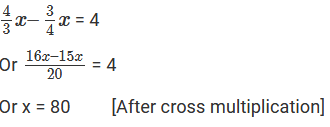Thus, the required number is 80.

Question: 2

The difference between the squares of two consecutive numbers is 31. Find the numbers.

Solution:

Let the numbers be x and x + 1.

According to the question,

(x + 1)2 – x2 = 31

or x2 + 2x + 1 – x2  = 31

or 2x = 31 – 1

or x = 30/2

or x = 15

Thus, the numbers are 15 and 16.

Question: 3

Find a number whose double is 45 greater than its half.

Solution:

Let the number be x.
According to the question,Question: 4

Find a number such that when 5 is subtracted from 5 times the number, the result is 4 more than twice the number.

Solution:

Let the number be x.
According to the question,

5x – 5 = 2x + 4

Or 5x – 2x = 4 + 5

Or 3x = 9

Or x = 9/3

Or x = 3

Thus, the number is 3.

Question: 5

A number whose fifth part increased by 5 is equal to its fourth part diminished by 5. Find the number.

Solution:

Let the number be x.
According to the question,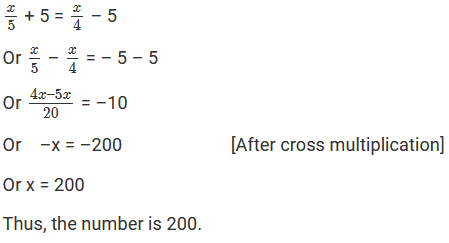Question: 6

A number consists of two digits whose sum is 9. If 27 is subtracted from the number, its digits are reversed. Find the number.

Solution:

Let the units digit be x.

Since, Sum of two digits = 9

∴ Tens digit = (9 – x)

∴ Original number = 10 × (9 – x) + x

Reversed number = 10x + (9 – x)

According to the question,

10 × (9 – x) + x – 27 = 10x + (9 – x)

Or 90 – 10x + x – 27 = 10x + 9 – x

Or 9x + 9x = 90 – 27 – 9

Or 18x = 54

Or x = 54/18 = 3

The number = 10 × (9 – 3) + 3 = 63

Question: 7

Divide 184 into two parts such that one-third of one part may exceed one-seventh of another part by 8.

Solution:

Let the first part of 184 be x.

Therefore, the other part will be (184 – x).

According to the question,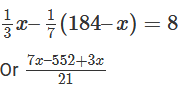10x – 552 = 168 [After cross multiplication]

Or 10x = 168 + 552

Or 10x = 720

Or x = 720/10 = 72

Thus, the parts of 184 are 72 and 112 (184 – 72 = 112).

Question: 8

The numerator of a fraction is 6 less than the denominator. If 3 is added to the numerator, the fraction is equal to 2/3. What is the original fraction equal to?

Solution:

Let the denominator of the fraction be x.

Therefore, the numerator will be (x – 6).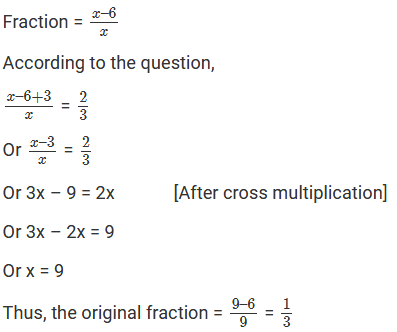Question: 9

A sum of Rs 800 is in the form of denominations of Rs 10 and Rs 20. If the total number of notes be 50, find the number of notes of each type.

Solution:

Let the number of Rs 10 notes be x.

Therefore, the number of Rs 20 notes will be (50 – x).

Value of Rs 10 notes = 10x

Value of Rs 20 notes = 20 (50 – x)

According to the question,

10x + 20(50 – x) = 800

Or 10x + 1000 – 20x = 800

Or 10x = 1000 – 800

Or x = 200/10 = 20

Therefore, Number of Rs 10 notes = 20

Number of Rs 20 notes = (50 – 20) = 30.

Question: 10

Seeta Devi has Rs 9 in fifty-paise and twenty five-paise coins. She has twice as many twenty-five paise coins as she has fifty-paise coins. How many coins of each kind does she have?

Solution:

Let the number of 50 paise coins be x.

Therefore, the number of 25 paise coins will be 2x.

Value of 50 paise coins = Rs. 0.5x

Value of 25 paise coins = Rs. 0.25 × 2x

According to the question,

0.5x + 0.25 × 2x = 9

or x = 9

∴ Number of fifty paise coins = 9

Number of twenty five paise coins = 2 × 9 = 18

Total number of coins = 9 + 18 = 27.

Question: 11

Sunita is twice as old as Ashima. If six years is subtracted from Ashima’s age and four years added to Sunita’s age, then Sunita will be four times Ashima’s age. How old were they two years ago?

Solution:

Let the age of Ashima be x years.

Therefore, the age of Sunita will be 2x years.

According to the question,

4(x – 6) = 2x + 4

or 4x – 24 = 2x + 4

or 4x – 2x = 4 + 24

or 2x = 28

or x = 14

∴ Age of Ashima = 14 years.

Age of Sunita = 2 × 14 = 28 years.

Question: 12

The ages of Sonu and Monti are in the ratio 7: 5. Ten years hence, the ratio of their ages will be 9: 7. Find their present ages.

Solution:

It is given that the ratio of the ages of Sonu and Monu is 7: 5.

Let the present ages of Sonu and Monu be 7x and 5x years.

After ten years:

Age of Sonu = 7x + 10 years

Age of Monu = 5x + 10 years

According to the question,

Or 49x + 70 = 45x + 90

Or 49x – 45x = 90 – 70

Or 4x = 20

Or x = 5

∴ Present age of Sonu = 7 × 5 = 35 years.

Present age of Monu = 5 × 5 = 25 years.

Question: 13

Five years ago a man was seven times as old as his son. Five years hence, the father will be three times as old as his son. Find their present ages.

Solution:

Five years ago:

Let the age of the son be x years.

Therefore, the age of the father will be 7x years.

∴ Present age of the son = (x + 5) years

Present age of the father = (7x + 5) years

After five years:

Age of the son = (x + 5 + 5) = (x + 10) years

Age of the father = (7x + 5 + 5) = (7x + 10) years

According to the question, 7x + 10 = 3 (x + 10)

or 7x – 3x = 30 – 10

or 4x = 20

or x = 5

∴ Present age of the son = (5 + 5) = 10 years.

Present age of the father = (7 × 5 + 5) = 40 years.

Question: 14

I am currently 5 times as old as my son. In 6 years time, I will be three times as old as he will be then. What are our ages now?

Solution:

Let the age of my son be x years.

Therefore, my age will be 5x years.

After 6 years:

Age of my son = (x + 6) years

My age = (5x + 6) years

According to the question,

5x + 6 = 3 (x + 6)

or 5x – 3x = 18 – 6

or 2x = 12

or x = 6

∴ Age of my son = 6 years.

My age = 5 × 6 = 30 years.

Question: 15

I have Rs 1000 in ten and five rupee notes. If the number of ten rupee notes that I have is ten more than the number of five rupee notes, how many notes do I have in each denomination?

Solution:

Let the number of five – rupee notes be x.

Therefore, the number of ten- rupee notes will be (x +10).

Now,

Value of five – rupee notes = Rs. 5x

Value of ten – rupee notes = Rs. 10 (x + 10)

According to the question,

5x + 10 (x + 10) = 1000

or 15x = 1000 – 100

or x = 90015 = 60

∴ Number of five – rupee notes = 60.

Number of ten – rupee notes = 60 + 10 = 70.

Question: 16

At a party, colas, squash and fruit juice were offered to guests. A fourth of the guests drank colas, a third drank squash, two-fifths drank fruit juice and just three did not drink anything. How many guests were in all?

Solution:

Let the total number of guests be x.

Therefore, the number of guests, who drank colas, would be (1/4)x.

The number of guests, who drank squash, would be (1/3)x.

The number of guests, who drank fruit juice, would be (2/5)x.

The number of guests, who did not drink, would be 3.

According to the question,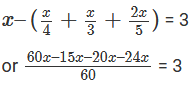or x = 180

Thus, total number of guests = 180.

Question: 17

There are 180 multiple choice questions in a test. If a candidate gets 4 marks for every correct answer and for every unattempted or wrongly answered question one mark is deducted from the total score of correct answers. If a candidate scored 450 marks in the test, how many questions did he answer correctly?

Solution:

Let the number of correctly answered questions be x.

Therefore, the number of unattempted or wrongly answered questions will be (180 – x).

According to the question,

4x – 1 (180 – 1) = 450

or 5x = 450 + 180

or x = 630/5 = 126

Thus, number of correctly answered questions = 126.

Number of unattempted or wrongly answered questions = 180 – 126 = 54.

Question: 18

A labourer is engaged for 20 days on the condition that he will receive Rs 60 for each day, he works and he will be fined Rs 5 for each day, he is absent. If he receives Rs 745 in all, for how many days he remained absent?

Solution:

Let the number of days for which the labourer is absent be x.

Therefore, the number of days for which he is present will be (20 - x).

Earnings = Rs. 60 (20 – x)

Fine = Rs. 5x

According to the question,

60(20 – x) – 5x = 745

or 1200 – 60x – 5x = 745

or 65x = 1200 — 745

or x = 455/65 = 7

Thus, the labourer was absent for 7 days.

Question: 19

Ravish has three boxes whose total weight is 60(1/2) kg. Box B weighs 3(1/2) kg more than box A and box C weighs 5(1/2) kg more than box B. Find the weight of box A.

Solution: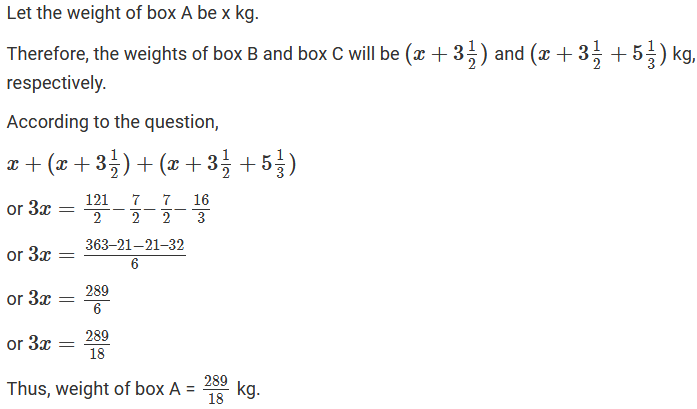Question: 20

The numerator of a rational number is 3 less than the denominator. If the denominator is increased by 5 and the numerator by 2, we get the rational number 1/2. Find the rational number.

Solution:

Let the denominator of the rational number be x.

∴ The numerator of the rational number will be x – 3.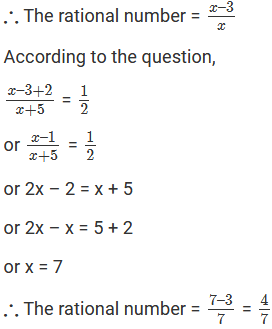Question: 21

In a rational number, twice the numerator is 2 more than the denominator. If 3 is added to each, the numerator and the denominator, the new fraction is 2/3. Find the original number.

Solution: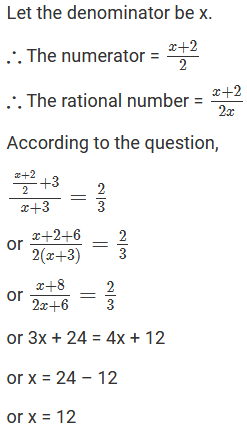Question: 22

The distance between two stations is 340 km. Two trains start simultaneously from these stations on parallel tracks to cross each other. The speed of one of them is greater than that of the other by 5 km/hr. If the distance between the two trains after 2 hours of their start is 30 km, find the speed of each train.

Solution:

Let, the speed of the first train be x km/h.

Then, the speed of the other train will be (x + 5) km/h.

2 hours after they started:

Distance of the first train from the starting point = 2x km

Distance of the other train from the starting point = 2 (x + 5) km

Now, 2(x + 5) + 2x + 30 = 340

or 4x + 10 + 30 = 340

or 4x = 340 – 40

or x = 300/4 = 75

∴ Speed of the first train = 75 km/h

Speed of the other train = (75 + 5) = 80 km/h

Question: 23

A steamer goes downstream from one point to another in 9 hours. It covers the same distance upstream in 10 hours. If the speed of the stream be 1 km/hr, find the speed of the steamer in still water and the distance between the ports.

Solution:

It is given that the speed of the stream is 1 km/h.

Let the speed of the steamer in still water be x km/h

∴ Downstream speed = (x + 1) km/h

Upstream speed = (x – 1) km/h

The downstream and upstream distances are same; therefore, we have:

9(x + 1) = 10(x – 1)

or 9x + 9 = 10x – 10

or x = 19

∴ Speed of the steamer in still water = 19 km/h.

Distance between the ports = 9 (19 + 1) = 180 km.

Question: 24

Bhagwanti inherited Rs 12000.00. She invested part of it at 10% and the rest at 12%. Her annual income from these investments is Rs 1280.00. How much did she invest at each rate?

Solution:

At the rate of 10%, let the investment by Bhagwanti be Rs. x.

Therefore, at the rate of 12%, the investment will be Rs. (12000 – x).

At the rate of 10%, her annual income = x × 10%

At the rate of 12%, her annual income = (12000 – x) × 12%

So,

x × 0.1 + 0.12 (12000 – x) = 1280

or 0.1 x – 0.12x = 1280 – 1440

or 0.02x = 160

or x = 8000

Thus, at the rate of 10%, she invested Rs. 8000 and at the rate of 12%, she invested Rs. 4000(12000 – 8000).

Question: 25

The length of a rectangle exceeds its breadth by 9 cm. If length and breadth are each increased by 3 cm, the area of the new rectangle will be 84 cm2 more than that of the given rectangle. Find the length and breadth of the given rectangle.

Solution:

Let the breadth of the rectangle be x cm.

Therefore, the length of the rectangle will be (x + 9) cm.

Area of the rectangle = x (x + 9) cm2.

If the length and breadth are increased by 3 cm each,

Area = (x + 3) (x + 9 + 3) cm2.

Now,

(x + 3) (x + 12) – x (x + 9) = 84

or x2 + 15x + 36 – x2 – 9x = 84

or 6x = 84 – 36

or x = 48/6 = 8.

Thus, breadth of the rectangle = 8 cm.

Length of the rectangle = (8 + 9) = 17 cm.

Question: 26

The sum of the ages of Anup and his father is 100. When Anup is as old as his father now, he will be five times as old as his son Anuj is now. Anuj will be eight years older than Anup is now, when Anup is as old as his father. What are their ages now?

Solution:

Let Anup’s age be x years.

Therefore, his father’s age will be (100 – x) years.

When Anup is as old as his father after (100 – 2x) years,Again, when Anup is as old as his father,

Anuj’s age = x + 8.

Now,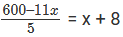or 600 – 11x = 5x + 40

or 16x = 560

or x = 35.

Thus, Anuj’s age = 35 years

Anuj’s father’s age = 100 – x = 100 – 35 = 65 years

Anuj’s age = x + 8 = 35 + 8 = 43 years

Question: 27

A lady went shopping and spent half of what she had on buying hankies and gave a rupee to a begger waiting outside the shop. She spent half of what was left of a lunch and followed that up with a two rupee tip. She spent half of the remaining amount on a book and three rupees on bus fare. When she reached home, she found that she had exactly one rupee left. How much money did she start with?

Solution:

Suppose, the lady started with x rupees.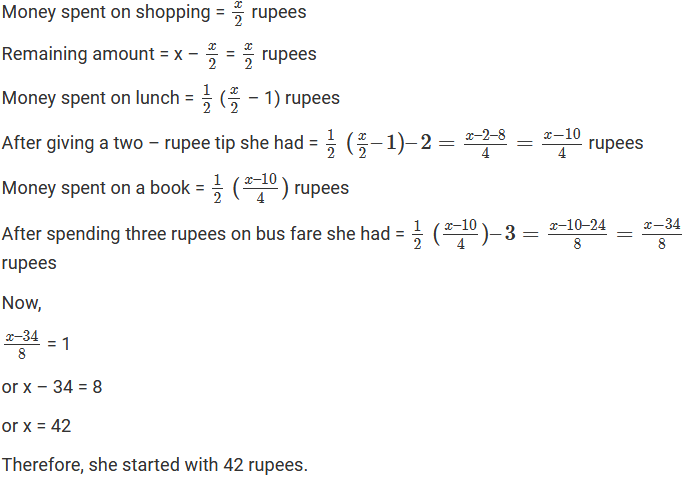### Course Features

• 728 Video Lectures
• Revision Notes
• Previous Year Papers
• Mind Map
• Study Planner
• NCERT Solutions
• Discussion Forum
• Test paper with Video Solution